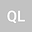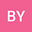Two regularity criteria of solutions to the liquid crystal flows
••• Qian Li,
• Baoquan Yuan
Qian Li
xinyang xueyuan

Corresponding Author:liqiang5412@163.com

Author ProfileBaoquan Yuan
Henan Polytechnic University
Author Profile## Abstract

In this paper, we derive two regularity criteria of solutions to the nematic liquid crystal flows. More precisely, we prove the local smooth solution $(u, d)$ is regular if and only if one of the following two conditions is satisfied: (i) $\nabla_{h} u_{h}\in L^{\frac{2p}{2p-3}}(0,T; L^{p}(\mathbb{R}^{3})),\ \partial_{3} d\in L^{\frac{2q}{q-3}}(0,T; L^{q}(\mathbb{R}^{3})),\ \frac{3}{2}< p\leq\infty,\ 3< q\leq\infty$; and (ii) \$\nabla_{h} u_{h}\in L^{q}(0,T; L^{p}(\mathbb{R}^{3})),\ \frac{3}{p}+\frac{2}{q}\leq 1, \ 3
19 Aug 2022Submitted to Mathematical Methods in the Applied Sciences
19 Aug 2022Assigned to Editor
19 Aug 2022Submission Checks Completed
13 Sep 2022Reviewer(s) Assigned
01 Jan 2023Review(s) Completed, Editorial Evaluation Pending
02 Jan 2023Editorial Decision: Accept